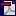http://www.deutschseite.de byCathy & Tom last updated: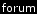Einheiten

 Länge (length)

If we want to express the length of something
or if we want to say how tall somebody is we use the units: Zentimeter [cm] and Meter [m].

How much is 1cm and how many cm are one m?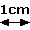100cm make 1m.
Every length above 100cm we express in meter.

To give you a better feeling how many xx cm is I prepared some examples with things
which have about the same size around the world: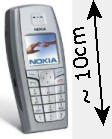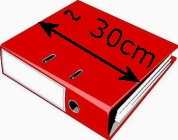conversion

1 inch = 2.54cm
1 foot = 30.48cm

Let's convert our both size into the cm/m- unit system: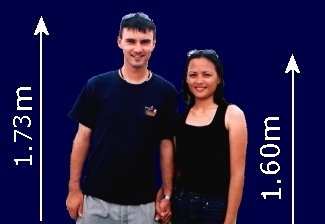Cathy You are 5 foot and 3 inches (5' 3'') tall. That are: 5 x 30.48cm + 3 x 2.54cm = 160.0cm So, you are 1.6m (=1m and 60cm) tall. Tom I'm 5 foot and 8 inches (5' 8'') tall. I'm: 5 x 30.48cm + 8 x 2.54cm = 172.7cm So, I'm 1.73m (=1m and 73cm) tall.

important expressions in connection with "Länge"

When you use these units you have to form a whole sentence. I want to give you some expressions:

 Deutsch Englisch Ich bin 1.60m groß. I'm 1.60m tall. Wie groß sind Sie? How tall are you? Der Schrank ist 2m hoch. The wardrobe 2m high. Wie lang ist der Tisch? How long is the table? Das Auto ist 1.5m breit. The car is 1.5m wide.

 Entfernung (distance)

If we want to express a distance between two places
we use the unit: Kilometer [km].

How much is 1km?

1000m make 1km.
Every distance above 1000m we express in Kilometer.The picture shows the city centre of Cagayan de Oro City.
The distance between the Sports Centre and the Limkit Kay Complex is about 1km.

The distance between the city centre (neverland cafe) and your mom's house in Agusan is 12km.

The distance between CDO and Manila (Rizal Park) is 780km.

conversion

1 mile = 1.61km

important expressions in connection with "Länge"

When you use this unit you have to form a whole sentence. I want to give you some expressions:

 Deutsch Englisch Die Entfernung zwischen Düren und CDO sind 11000km. The distance between Düren and CDO is 11000km. Wie weit ist es bis nach Manila? How far is it to Manila?

 Gewicht (weight)

If we want to express the weight of something or somebody
we use the units: Gramm [g] and Kilogramm [kg].

How many g are one kg?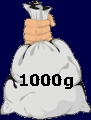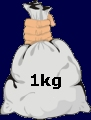1000g make 1kg.
Every weight above 1000g we express in Kilogramm.

conversion

1 pound = 454g
1 pound = 0.45kg

To make it easier you could say: Divide you weight in pounds by 2 and you get it in Kilogramm.

Let's convert our both weight into the g/kg- unit system: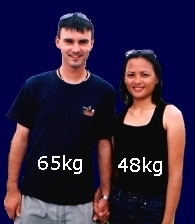Cathy You've got 105 lb. Converted into kg: 105 lb x 0.454 = 47.7kg So, you've got about 48kg. Tom I've got 143 lb. Converted into kg: 143 lb x 0.454 = 64.9kg So, I've got about 65kg.

important expressions in connection with "Gewicht"

When you use these units you have to form a whole sentence. I want to give you some expressions:

 Deutsch Englisch Ich wiege 48kg. I weigh 48kg. Wieviel wiegen Sie? What do you weigh? Wie schwer sind Sie? How heavy are you?

 Temperatur (temperature)

If we want to express the temperature
we use the unit: Grad Celsius [°C].

How warm or cold are xx °C?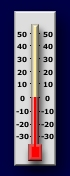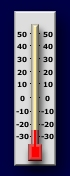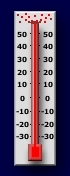1 2 3 4 5 6

 no. comment 1 25°C: Is the average temperature during the rainy season in the Philippines.I consdider 25°C as the perfect temperature to feel comfortable. 2 36°C: Is the average temperature during the hot dry season in the Philippines.36°C is really too warm, especially if you try to sleep without aircon. 3 8°C: Is the average temperature in a fridge. That's the temperature in Germany in fall and during sunny winter days. 4 0°C: Is the temperature when water frizes and becomes ice. That's a normal temperature in Germany during the winter. 5 -25°C: Is the lowest temperature I've ever experienced. It's damn cold and you shouldn't stay too long outside. 6 100°C: Is the tempereature when water starts boiling.

conversion

The conversion from °Fahrenheit into °C is a bit complicate. You have to use the following formula:
°C = (°Fahrenheit - 32) x 5/9

examples

80 °Fahrenheit = (80-32) x 5/9 = 26.7°C
20 °Fahrenheit = (20-32) x 5/9 = -6.7°C
0 °Fahrenheit = (0-32) x 5/9 = -17.8°C

important expressions in connection with "Temperatur"

When you use this unit you have to form a whole sentence. I want to give you some expressions:

 Deutsch Englisch Es ist 20° warm. It's 20° warm. Wir haben heute 30°. We've got 30° today. Wie warm ist es? How warm is it?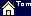Einheiten (5 pages)